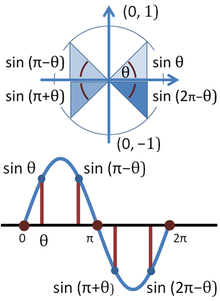# Statistics - (Periodicity|Periodic phenomena|Cycle)

Periodic phenomena such as:

• sound and light waves,
• the position and velocity of harmonic oscillators,
• sunlight intensity and day length,
• and average temperature variations throughout the year. See Time Serie - Seasonality (Cycle detection)

## Model

Trigonometric functions are important in modeling periodic phenomena:

All periodic functions can be exactly represented by Fourier Series

A function is periodic, with fundamental period T, if the following is true for all t: $$f(t+T)=f(t)$$

Recommended PagesData Mining - Data Mining - (Data|Knowledge) Discovery - Statistical Learning

Data Mining can be defined as the automatic or semiautomatic task of extracting previously unknown information from a large quantity of data. Data mining try to discover in data unknown: unexpected...Data Mining - Signal (Wanted Variation)

Information from all past experience can be divided into two groups: information that is relevant for the future (“signal”). pattern information that is irrelevant (“noise”). In the real...Time Serie - Seasonality (Cycle detection)

Seasonality is a cycle in time serie. The season is used a discreet regression variable and code it as dummy variables). For instance: 1 if it's the season 0 if it's not the season If you...Trigonometry - (Sine|Sinus)

Sine is the function of an angle (trigonometric function). The word comes from the Latin sinus for gulf or bay, since, given a unit circle, it is the side of the triangle on which the angle opens. ...Trigonometry - Fourier Series (Fourier Transform for periodic functions)

The Fourier Series breaks down a periodic function into the sum of sinusoidal functions. A Fourier series is a way to represent a wave-like function (like a square wave) as the sum of simple sine waves....Trigonometry - Fourier Transform

The Fourier transform decomposes a function of time (e.g., a periodic signal) into the frequencies that make it up, similarly to how a musical chord can be expressed as the amplitude (or loudness) of its...Trigonometry - Function

Trigonometric functions are function of an angle. Trigonometric functions are important in the modeling of periodic phenomena. List: sine, cosine, tangent Trigonometric_functionsWhat is a Pattern ?

A pattern means that the data (visual or not) are correlated that they have a relationship and that they are predictable. When you have a lack of pattern, you have true randomness When you find a pattern,...